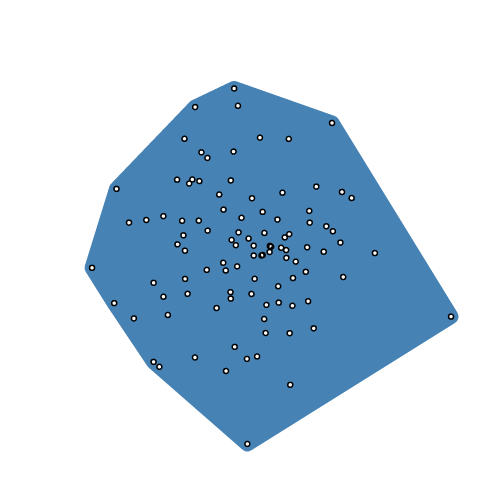Não Posso Mais

npm

d3-polygon3.0.1 • Public • Published

d3-polygon

This module provides a few basic geometric operations for two-dimensional polygons. Each polygon is represented as an array of two-element arrays [​[x1, y1], [x2, y2], …], and may either be closed (wherein the first and last point are the same) or open (wherein they are not). Typically polygons are in counterclockwise order, assuming a coordinate system where the origin ⟨0,0⟩ is in the top-left corner.

Installing

If you use npm, npm install d3-polygon. You can also download the latest release on GitHub. For vanilla HTML in modern browsers, import d3-polygon from Skypack:

<script type="module">

import {polygonHull} from "https://cdn.skypack.dev/d3-polygon@3";

const hull = polygonHull(points);

</script>

For legacy environments, you can load d3-polygon’s UMD bundle from an npm-based CDN such as jsDelivr; a d3 global is exported:

<script src="https://cdn.jsdelivr.net/npm/d3-polygon@3"></script>
<script>

const hull = d3.polygonHull(points);

</script>

API Reference

# d3.polygonArea(polygon) <>

Returns the signed area of the specified polygon. If the vertices of the polygon are in counterclockwise order (assuming a coordinate system where the origin ⟨0,0⟩ is in the top-left corner), the returned area is positive; otherwise it is negative, or zero.

# d3.polygonCentroid(polygon) <>

Returns the centroid of the specified polygon.

# d3.polygonHull(points) <>Returns the convex hull of the specified points using Andrew’s monotone chain algorithm. The returned hull is represented as an array containing a subset of the input points arranged in counterclockwise order. Returns null if points has fewer than three elements.

# d3.polygonContains(polygon, point) <>

Returns true if and only if the specified point is inside the specified polygon.

# d3.polygonLength(polygon) <>

Returns the length of the perimeter of the specified polygon.

Keywords

Install

npm i d3-polygon

Repository

github.com/d3/d3-polygon

Homepage

d3js.org/d3-polygon/

1,144,563

3.0.1